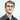# Advent of Code 2019 - Day 8

December 08, 2019

## Day 8: Space Image Format

### Part 1 - Implement the basics

Images are sent as a series of digits that each represent the color of a single pixel. The digits fill each row of the image left-to-right, then move downward to the next row, filling rows top-to-bottom until every pixel of the image is filled.

Each image actually consists of a series of identically-sized layers that are filled in this way. So, the first digit corresponds to the top-left pixel of the first layer, the second digit corresponds to the pixel to the right of that on the same layer, and so on until the last digit, which corresponds to the bottom-right pixel of the last layer.

To make sure the image wasn’t corrupted during transmission, the Elves would like you to find the layer that contains the fewest 0 digits. On that layer, what is the number of 1 digits multiplied by the number of 2 digits?

``````func main() {
w, h := 25, 6
line := lines
layers := [][][]int{}
for i := 0; i < len(line); i += w * h {
layer := [][]int{}
row := []int{}
for j := 0; j < w*h; j++ {
value, err := strconv.Atoi(string(line[i+j]))
check(err)
row = append(row, value)
if len(row) == w {
layer = append(layer, row)
row = []int{}
}
}
layers = append(layers, layer)
}
min0 := 9999999
minLayer := 0
count1 := []int{}
count2 := []int{}
for i:=0;i<len(layers);i++ {
count0 := 0
count1[i] = 0
count2[i] = 0
for _, row := range layers[i] {
for _, v := range row {
if v == 0 {
count0++
} else {
if(v == 1){
count1[i]++
}
if(v == 2){
count2[i]++
}
}
}
}
if count0 < min0 {
min0 = count0
minLayer = i
}
}
fmt.Println(count1[minLayer] * count2[minLayer])
}``````

Here we construct the arrays for each layer, checking the number of zeroes, ones and twos. After checking each layer we return the results from the layer with the least zeroes.

### Part 2 - Extract the BIOS password!

Now that we are happy the image decoding is working properly we need to handle stacking the layers (in reverse order) to produce the resulting image.

0 is a black pixel 1 is a white pixel 2 is a transparent pixel

``````func stacker(layers [][][]int) int {
result := int{}
for i := 0; i < len(result); i++ {
for j := 0; j < len(result[i]); j++ {
for k := len(layers) - 1; k >= 0; k-- {
newV := layers[k][i][j]
if newV != 2 {
result[i][j] = newV
}

}
}
}
return result
}

func main() {
w, h := 25, 6
line := lines
layers := [][][]int{}
for i := 0; i < len(line); i += w * h {
layer := [][]int{}
row := []int{}
for j := 0; j < w*h; j++ {
value, err := strconv.Atoi(string(line[i+j]))
check(err)
row = append(row, value)
if len(row) == w {
layer = append(layer, row)
row = []int{}
}
}
layers = append(layers, layer)
}
result := stacker(layers)
for _, row := range result {
for _, v := range row {
if v == 0 {
fmt.Printf(" ")
} else {
fmt.Printf(".")
}
}
fmt.Printf("\n")
}
}``````

The stacker function checks for transparent pixels first as these have no effect on the underlying pixel (i.e black stays black, white stays white). For other results we replace the pixel with the overlying colour i.e 0 replaces 1 or 1 replaces 0.

We then print the resulting stacked array, replacing 0 with a space and 1 with a . to make for a clear printing. The result of my BIOS password can be seen below.

### Conclusion

A fun detour after yesterday’s difficult part 2, with a cool bit of visual output as a result!

If you have any feedback on my Go code, I’d be happy to hear it!

Github RepoWritten by Daniel Betteridge who lives and works in Perth, WA building useful things.